# SSAT Middle Level Math : Distributive Property

## Example Questions

### Example Question #31 : Distributive Property

Use the distributive property to express the sum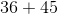as the multiple of a sum of two whole numbers with no common factor.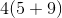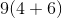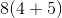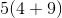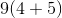Explanation:

The distributive property can be used to rewrite an expression. When we use this property we will identify and pull out the greatest common factor of each of the addends. Then we can create a quantity that represents the sum of two whole numbers with no common factor multiplied by their greatest common factor.In this case, the greatest common factor shared by each number is: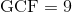After we reduce each addend by the greatest common factor we can rewrite the expression:### Example Question #32 : Distributive Property

Use the distributive property to express the sum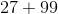as the multiple of a sum of two whole numbers with no common factor.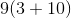None of these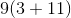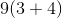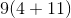Explanation:

The distributive property can be used to rewrite an expression. When we use this property we will identify and pull out the greatest common factor of each of the addends. Then we can create a quantity that represents the sum of two whole numbers with no common factor multiplied by their greatest common factor.In this case, the greatest common factor shared by each number is:After we reduce each addend by the greatest common factor we can rewrite the expression:### Example Question #33 : Distributive Property

Use the distributive property to express the sum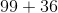as the multiple of a sum of two whole numbers with no common factor.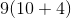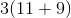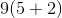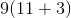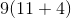Explanation:

The distributive property can be used to rewrite an expression. When we use this property we will identify and pull out the greatest common factor of each of the addends. Then we can create a quantity that represents the sum of two whole numbers with no common factor multiplied by their greatest common factor.In this case, the greatest common factor shared by each number is:After we reduce each addend by the greatest common factor we can rewrite the expression:### Example Question #34 : Distributive Property

Use the distributive property to express the sum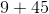as the multiple of a sum of two whole numbers with no common factor.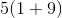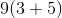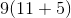None of theseExplanation:

The distributive property can be used to rewrite an expression. When we use this property we will identify and pull out the greatest common factor of each of the addends. Then we can create a quantity that represents the sum of two whole numbers with no common factor multiplied by their greatest common factor.In this case, the greatest common factor shared by each number is:After we reduce each addend by the greatest common factor we can rewrite the expression: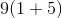### Example Question #22 : Find Greatest Common Factor And Least Common Multiple: Ccss.Math.Content.6.Ns.B.4

Use the distributive property to express the sum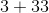as the multiple of a sum of two whole numbers with no common factor.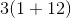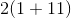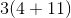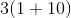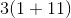Explanation:

The distributive property can be used to rewrite an expression. When we use this property we will identify and pull out the greatest common factor of each of the addends. Then we can create a quantity that represents the sum of two whole numbers with no common factor multiplied by their greatest common factor.In this case, the greatest common factor shared by each number is: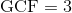After we reduce each addend by the greatest common factor we can rewrite the expression:### Example Question #36 : How To Find The Distributive Property

Use the distributive property to express the sum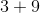as the multiple of a sum of two whole numbers with no common factor.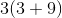None of these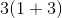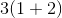Explanation:

The distributive property can be used to rewrite an expression. When we use this property we will identify and pull out the greatest common factor of each of the addends. Then we can create a quantity that represents the sum of two whole numbers with no common factor multiplied by their greatest common factor.In this case, the greatest common factor shared by each number is:After we reduce each addend by the greatest common factor we can rewrite the expression:### Example Question #35 : Distributive Property

Use the distributive property to express the sum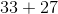as the multiple of a sum of two whole numbers with no common factor.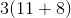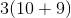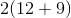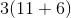Explanation:

The distributive property can be used to rewrite an expression. When we use this property we will identify and pull out the greatest common factor of each of the addends. Then we can create a quantity that represents the sum of two whole numbers with no common factor multiplied by their greatest common factor.In this case, the greatest common factor shared by each number is:After we reduce each addend by the greatest common factor we can rewrite the expression:### Example Question #31 : How To Find The Distributive Property

Use the distributive property to express the sum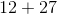as the multiple of a sum of two whole numbers with no common factor.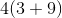None of these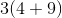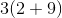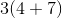Explanation:

The distributive property can be used to rewrite an expression. When we use this property we will identify and pull out the greatest common factor of each of the addends. Then we can create a quantity that represents the sum of two whole numbers with no common factor multiplied by their greatest common factor.In this case, the greatest common factor shared by each number is:After we reduce each addend by the greatest common factor we can rewrite the expression:### Example Question #32 : How To Find The Distributive Property

Use the distributive property to express the sum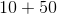as the multiple of a sum of two whole numbers with no common factor.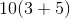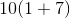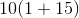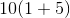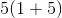Explanation:

The distributive property can be used to rewrite an expression. When we use this property we will identify and pull out the greatest common factor of each of the addends. Then we can create a quantity that represents the sum of two whole numbers with no common factor multiplied by their greatest common factor.In this case, the greatest common factor shared by each number is: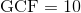After we reduce each addend by the greatest common factor we can rewrite the expression:### Example Question #31 : How To Find The Distributive Property

Use the distributive property to express the sum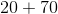as the multiple of a sum of two whole numbers with no common factor.

None of these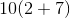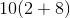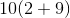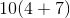Explanation:

The distributive property can be used to rewrite an expression. When we use this property we will identify and pull out the greatest common factor of each of the addends. Then we can create a quantity that represents the sum of two whole numbers with no common factor multiplied by their greatest common factor.In this case, the greatest common factor shared by each number is:After we reduce each addend by the greatest common factor we can rewrite the expression:### All SSAT Middle Level Math Resources